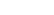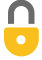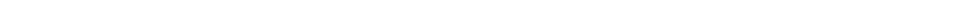Problem 11
Q
1. How much energy is required to bring a 1.0-L pot of water at $20 ^\circ \textrm{C}$ to $100 ^\circ \textrm{C}$?
2. For how long could this amount of energy run a 60-W lightbulb?A
1. $3.3 \times 10^5 \textrm{ J}$
2. $93 \textrm{ min}$In order to watch this solution you need to have a subscription.VIDEO TRANSCRIPT

This is Giancoli Answers with Mr. Dychko. The heat gained by this pot of water is going to be the mass of water times specific heat capacity times change in temperature. And the mass will be the volume times density. And the volume is 1.0 liters times 1 kilogram for every liter, density of water, times 4,186 joules per kilogram Celsius degree times the change in temperature, 100 degree Celsius minus 20. And that gives 3.3 times 10 to the 5 joules. And the amount of time that this amount of energy could power a 60 watt bulb. Well, we'll figure that out by knowing that power is energy divided by time. And then we'll solve this for t by multiplying both sides by t over p. Then the left side were left with time and on the right side we have p in the bottom. And that's energy divided by power. So, that's 3.3488 times 10 to the 5 joules divided by 60 watts which is about 93 minutes after you convert the 5,581 seconds times by 1 minute for every 60 seconds. 93 minutes, the 60 watt bulb could be powered.

Giancoli Answers, including solutions and videos, is copyright © 2009-2023 Shaun Dychko, Vancouver, BC, Canada. Giancoli Answers is not affiliated with the textbook publisher. Book covers, titles, and author names appear for reference purposes only and are the property of their respective owners. Giancoli Answers is your best source for the 7th and 6th edition Giancoli physics solutions.